Test: Theorems of Integral Calculus- 2

# Test: Theorems of Integral Calculus- 2

Test Description

## 20 Questions MCQ Test GATE Mechanical (ME) 2023 Mock Test Series | Test: Theorems of Integral Calculus- 2

Test: Theorems of Integral Calculus- 2 for Civil Engineering (CE) 2023 is part of GATE Mechanical (ME) 2023 Mock Test Series preparation. The Test: Theorems of Integral Calculus- 2 questions and answers have been prepared according to the Civil Engineering (CE) exam syllabus.The Test: Theorems of Integral Calculus- 2 MCQs are made for Civil Engineering (CE) 2023 Exam. Find important definitions, questions, notes, meanings, examples, exercises, MCQs and online tests for Test: Theorems of Integral Calculus- 2 below.
Solutions of Test: Theorems of Integral Calculus- 2 questions in English are available as part of our GATE Mechanical (ME) 2023 Mock Test Series for Civil Engineering (CE) & Test: Theorems of Integral Calculus- 2 solutions in Hindi for GATE Mechanical (ME) 2023 Mock Test Series course. Download more important topics, notes, lectures and mock test series for Civil Engineering (CE) Exam by signing up for free. Attempt Test: Theorems of Integral Calculus- 2 | 20 questions in 60 minutes | Mock test for Civil Engineering (CE) preparation | Free important questions MCQ to study GATE Mechanical (ME) 2023 Mock Test Series for Civil Engineering (CE) Exam | Download free PDF with solutions
 1 Crore+ students have signed up on EduRev. Have you?
Test: Theorems of Integral Calculus- 2 - Question 1

### A function is given by f(t) = sin2t + cos 2t. Which of the following is true?

Detailed Solution for Test: Theorems of Integral Calculus- 2 - Question 1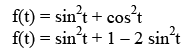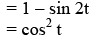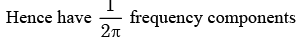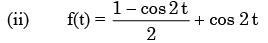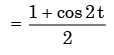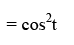Test: Theorems of Integral Calculus- 2 - Question 2

### Following are the values of a function y(x) : y(-1) = 5, y(0), y(1)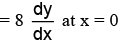as per Newton’s central  difference scheme is:

Detailed Solution for Test: Theorems of Integral Calculus- 2 - Question 2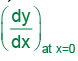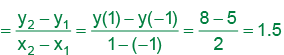Test: Theorems of Integral Calculus- 2 - Question 3

###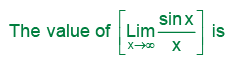Detailed Solution for Test: Theorems of Integral Calculus- 2 - Question 3

the squeeze theorem for this. Recall that sinx is only defined on −1≤sinx≤1. Therefore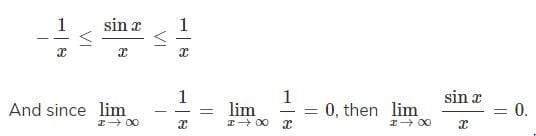Test: Theorems of Integral Calculus- 2 - Question 4

The function f(x) = |x+1| on the interval [-2, 0]

Detailed Solution for Test: Theorems of Integral Calculus- 2 - Question 4

f(x ) = x+ 1

f is continuous in [−2, 0]

but not differentiable at

x =−1 because we can draw

infinite number of tangents at x = −1

Test: Theorems of Integral Calculus- 2 - Question 5

If y=|x| for x<0 and y=x for x ≥ 0, then

Detailed Solution for Test: Theorems of Integral Calculus- 2 - Question 5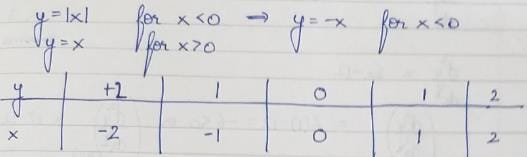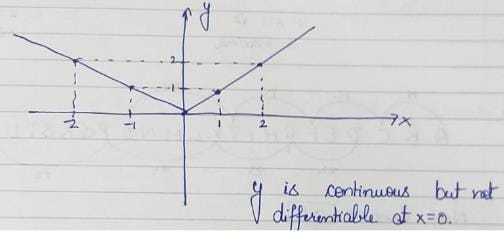Test: Theorems of Integral Calculus- 2 - Question 6

What is the derivative of f(x) = |x| at x = 0?

Detailed Solution for Test: Theorems of Integral Calculus- 2 - Question 6

f( x ) = x .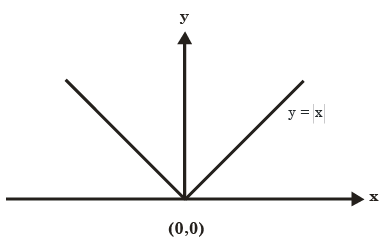At x = 0, we can draw infinitely many tangents at x=0.So limit does not exists.

Test: Theorems of Integral Calculus- 2 - Question 7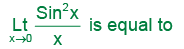Detailed Solution for Test: Theorems of Integral Calculus- 2 - Question 7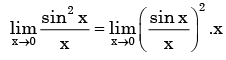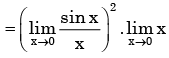= 1.0= 1

Test: Theorems of Integral Calculus- 2 - Question 8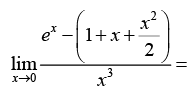Detailed Solution for Test: Theorems of Integral Calculus- 2 - Question 8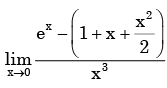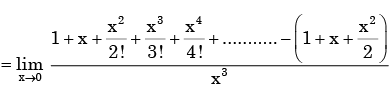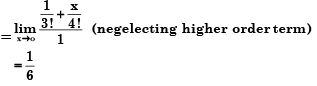Test: Theorems of Integral Calculus- 2 - Question 9

The function Y=| 2-3x |

Test: Theorems of Integral Calculus- 2 - Question 10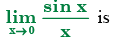Test: Theorems of Integral Calculus- 2 - Question 11

The function f(x) = x3 - 6x2 + 9x + 25 has

Detailed Solution for Test: Theorems of Integral Calculus- 2 - Question 11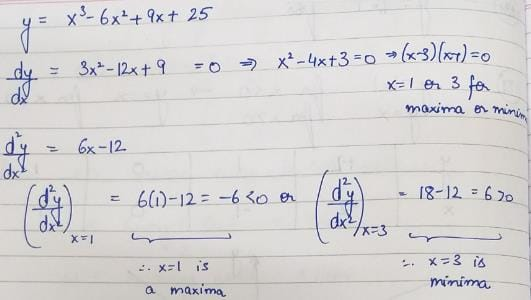Test: Theorems of Integral Calculus- 2 - Question 12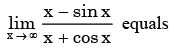Detailed Solution for Test: Theorems of Integral Calculus- 2 - Question 12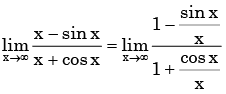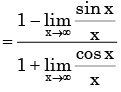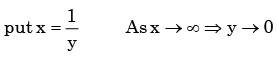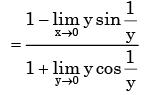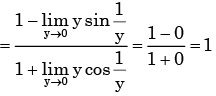Test: Theorems of Integral Calculus- 2 - Question 13

The value of the integral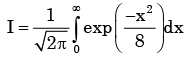is

Detailed Solution for Test: Theorems of Integral Calculus- 2 - Question 13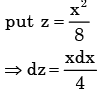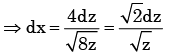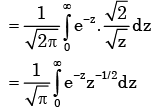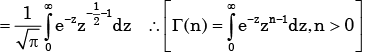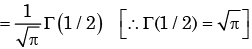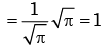Test: Theorems of Integral Calculus- 2 - Question 14

The following plot shows a function y which varies linearly with x. The value of the integral I =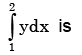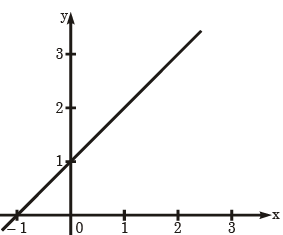Detailed Solution for Test: Theorems of Integral Calculus- 2 - Question 14

Here the points (0,1) and (-1,0) are on the time

∴The equn of the line is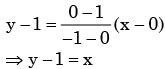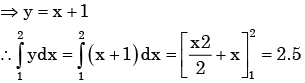Test: Theorems of Integral Calculus- 2 - Question 15

The value of the integral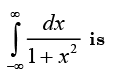Detailed Solution for Test: Theorems of Integral Calculus- 2 - Question 15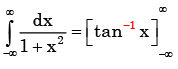Test: Theorems of Integral Calculus- 2 - Question 16

The length of the curve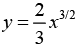between x = 0 and x = 1 is

Detailed Solution for Test: Theorems of Integral Calculus- 2 - Question 16

Length of  the wire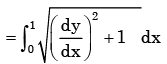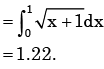Test: Theorems of Integral Calculus- 2 - Question 17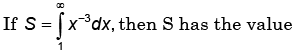Detailed Solution for Test: Theorems of Integral Calculus- 2 - Question 17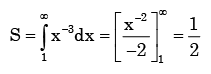Test: Theorems of Integral Calculus- 2 - Question 18

A continuous-time system is described by y (t) = e − x (t) where y (t) is the output and x (t) is the input. y(t) is bounded.

Detailed Solution for Test: Theorems of Integral Calculus- 2 - Question 18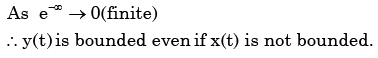Test: Theorems of Integral Calculus- 2 - Question 19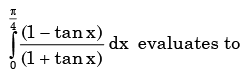Detailed Solution for Test: Theorems of Integral Calculus- 2 - Question 19

Since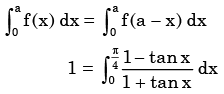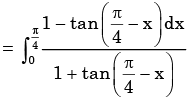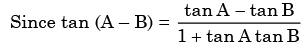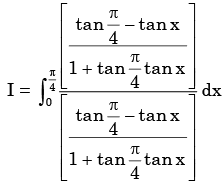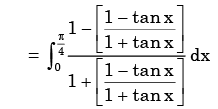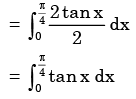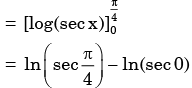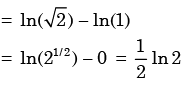Test: Theorems of Integral Calculus- 2 - Question 20

The value of the quantity P, where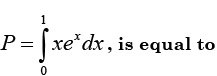Detailed Solution for Test: Theorems of Integral Calculus- 2 - Question 20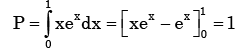## GATE Mechanical (ME) 2023 Mock Test Series

27 docs|243 tests
 Use Code STAYHOME200 and get INR 200 additional OFF Use Coupon Code
Information about Test: Theorems of Integral Calculus- 2 Page
In this test you can find the Exam questions for Test: Theorems of Integral Calculus- 2 solved & explained in the simplest way possible. Besides giving Questions and answers for Test: Theorems of Integral Calculus- 2, EduRev gives you an ample number of Online tests for practice

## GATE Mechanical (ME) 2023 Mock Test Series

27 docs|243 tests